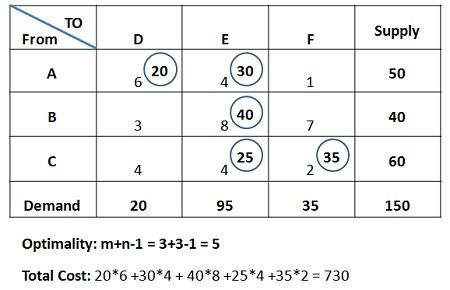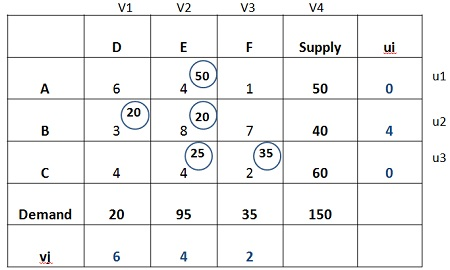# Testing For Optimality

Once the feasible solution is obtained, the next step is to check whether it is optimum or not. There are two methods used for testing the optimality:

• Stepping-stone Method
• Modified Distribution Method (MODI)

### STEPPING STONE METHOD

The Stepping Stone Method is used to check the optimality of the initial feasible solution determined by using any of the method Viz. North-West Corner, Least Cost Method or Vogel’s Approximation Method. Thus, the stepping stone method is a procedure for finding the potential of any non-basic variables (empty cells) in terms of the objective function.

Through stepping stone method, we determine that what effect on the transportation cost would be in case one unit is assigned to the empty cell. With the help of this method, we come to know whether the solution is optimal or not.

The series of steps are involved in checking the optimality of the initial feasible solution using the stepping stone method:

1. The prerequisite condition to solve for the optimality is to ensure that the number of occupied cells is exactly equal to m+n-1, where ‘m’ is the number of rows, while ‘n’ is equal to the number of columns.
2. Firstly, the empty cell is selected and then the closed path is created which starts from the unoccupied cell and returns to the same unoccupied cell, called as a “closed loop”. For creating a closed loop the following conditions should be kept in mind:
• In a closed loop, cells are selected in a sequence such that one cell is unused/unoccupied, and all other cells are used/occupied.
• A pair of Consecutive used cells lies either in the same row or the same column.
• No three consecutive occupied cells can either be in the same row or column.
• The first and last cells in the closed loop lies either in the same row or column.
• Only horizontal and vertical movement is allowed.
• Once the loop is created, assign “+” or “–“ sign alternatively on each corner cell of the loop, but begin with the “+” sign for the unoccupied cell.
• Repeat these steps again until all the unoccupied cells get evaluated.
• Now, if all the computed changes are positive or are equal to or greater than zero, then the optimal solution has been reached.
• But in case, if any, value comes to be negative, then there is a scope to reduce the transportation cost further. Then, select that unoccupied cell which has the most negative change and assign as many units as possible. Subtract the unit that added to the unoccupied cell from the other cells with a negative sign in a loop, to balance the demand and supply requirements.

Example, suppose the following matrix shows the initial feasible solution and stepping stone method is adopted to check its optimality:With the new matrix so formed, again the empty cells will be evaluated through a loop formation and signs will be assigned accordingly. The cell with the highest opportunity cost will be assigned the units, and this process will repeat until the best optimum solution is obtained or the opportunity cost of all the unoccupied cells comes to be negative.

### MODIFIED DISTRIBUTION METHOD

Definition: The Modified Distribution Method or MODI is an efficient method of checking the optimality of the initial feasible solution.

The concept of MODI can be further comprehended through an illustration given below:

• Initial basic feasible solution is given below:• Now, calculate the values of ui and vj by using the equation:
ui+vj = Cij
Substituting the value of u1 as 0

U1+V1 = C11, 0+V1 = 6 or V1 = 6
U1 +V2 = C12, 0+V2 = 4 or V2 = 4
U2+V2 = C22, U2+4 = 8 or U2 = 4
U3+ V2 = C32, U3+4 = 4 or U3 = 0
U3+V3 = C33, 0+V3 = 2 or V3 =2• Next step is to calculate the opportunity cost of the unoccupied cells (AF, BD, BF, CD) by using the following formula:
Cij – (ui+Vi)

AF = C13 – (U1+V3),  1- (0+2) = -1 or 1
BD = C21 – (U2+v1),   3- (4+6) = -7 or 7
BF = C23 – (U2+V3),   7- (4+2) = 1 or -1
CD = C31- (U3+V1),    4- (0+6) = -2 or 2
• Choose the largest positive opportunity cost, which is 7 and draw a closed path, as shown in the matrix below. Start from the unoccupied cell and assign “+” or “–“sign alternatively. Therefore, The most favored cell is BD, assign as many units as possible.• The matrix below shows the maximum allocation to the cell BD, and that number of units are added to the cell with a positive sign and subtracted from the cell with a negative sign.• Again, repeat the steps from 1 to 4 i.e. find out the opportunity costs for each unoccupied cell and assign the maximum possible units to the cell having the largest opportunity cost. This process will go on until the optimum solution is reached.

The Modified distribution method is an improvement over the stepping stone method since; it can be applied more efficiently when a large number of sources and destinations are involved, which becomes quite difficult or tedious in case of stepping stone method.

Modified distribution method reduces the number of steps involved in the evaluation of empty cells, thereby minimizes the complexity and gives a straightforward computational scheme through which the opportunity cost of each empty cell can be determined.

## One thought on “Testing For Optimality”

error: Content is protected !!